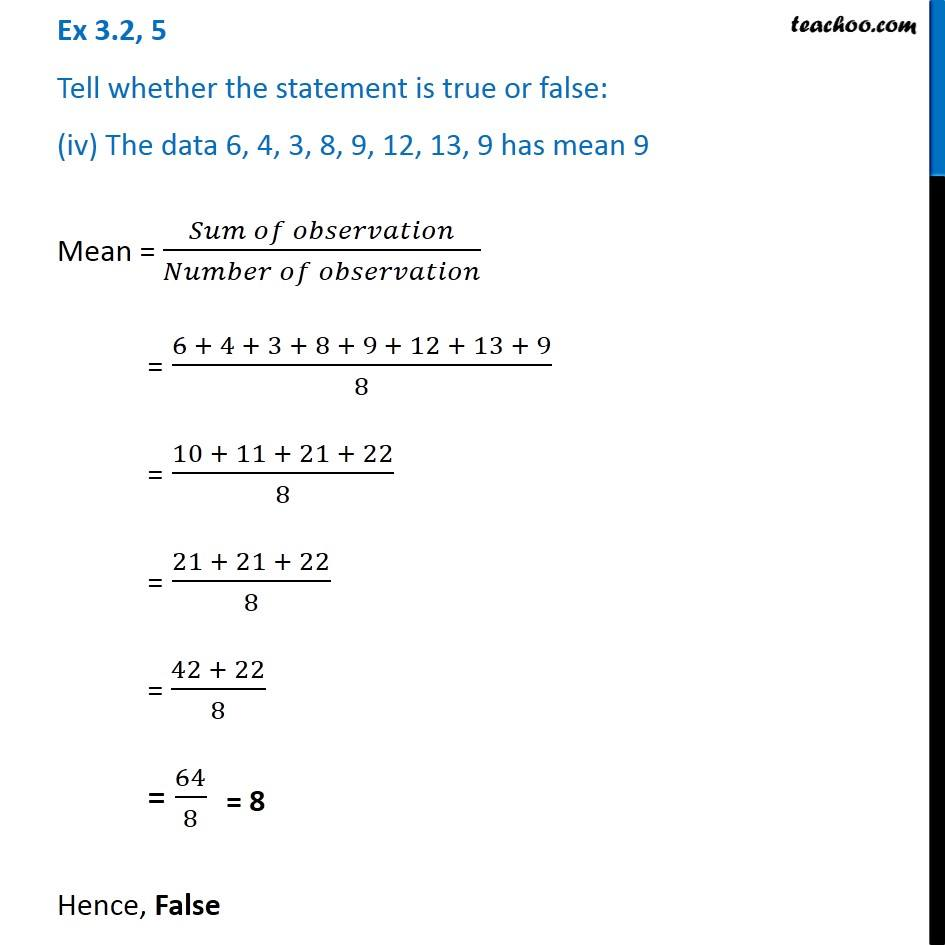Ex 3.2

Chapter 3 Class 7 Data Handling
Serial order wiseLearn in your speed, with individual attention - Teachoo Maths 1-on-1 Class

### Transcript

Ex 3.2, 5 Tell whether the statement is true or false: (iv) The data 6, 4, 3, 8, 9, 12, 13, 9 has mean 9 Mean = (𝑆𝑢𝑚 𝑜𝑓 𝑜𝑏𝑠𝑒𝑟𝑣𝑎𝑡𝑖𝑜𝑛)/(𝑁𝑢𝑚𝑏𝑒𝑟 𝑜𝑓 𝑜𝑏𝑠𝑒𝑟𝑣𝑎𝑡𝑖𝑜𝑛) = (6 + 4 + 3 + 8 + 9 + 12 + 13 + 9)/8 = (10 + 11 + 21 + 22)/8 = (21 + 21 + 22)/8 = (42 + 22)/8 = 64/8 Hence, False